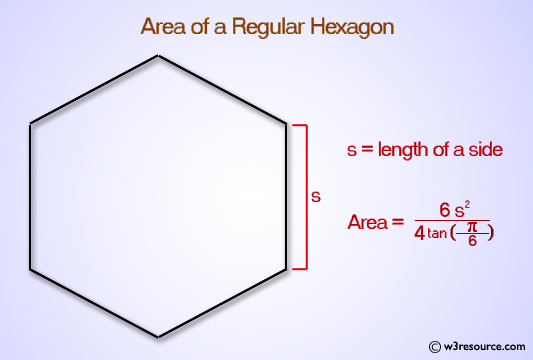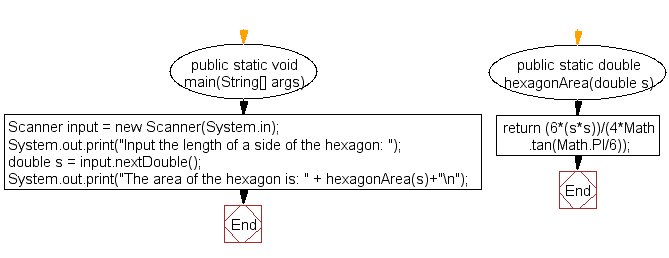﻿ Java exercises: Compute the area of a hexagon - w3resource# Java Exercises: Compute the area of a hexagon

## Java Basic: Exercise-34 with Solution

Write a Java program to compute the area of a hexagon.

Test Data:
Input the length of a side of the hexagon: 6

Pictorial Presentation: Area of HexagonSample Solution:

Java Code:

``````import java.util.Scanner;
public class Exercise34 {
public static void main(String[] args) {
Scanner input = new Scanner(System.in);
System.out.print("Input the length of a side of the hexagon: ");
double s = input.nextDouble();
System.out.print("The area of the hexagon is: " + hexagonArea(s)+"\n");
}
public static double hexagonArea(double s) {
return (6*(s*s))/(4*Math.tan(Math.PI/6));
}
}
```
```

Sample Output:

```Input the length of a side of the hexagon: 6
The area of the hexagon is: 93.53074360871938
```

Flowchart:Java Code Editor:

What is the difficulty level of this exercise?

Test your Programming skills with w3resource's quiz.

﻿

## Java: Tips of the Day

countOccurrences

Counts the occurrences of a value in an array.

Use Arrays.stream().filter().count() to count total number of values that equals the specified value.

```public static long countOccurrences(int[] numbers, int value) {
return Arrays.stream(numbers)
.filter(number -> number == value)
.count();
}
```

Ref: https://bit.ly/3kCAgLb